# How to Calculate and Solve for the Reaction at the Inner Wheel of a Vehicle moving along a Level Circular Path | The Calculator Encyclopedia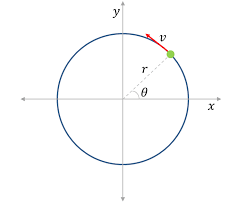The image represents reaction at the inner wheel of a vehicle moving along a level circular path.

To compute for the reaction, six essential parameters are needed and these parameters are Mass of the Vechicle (m), Acceleration due to Gravity (g), Velocity of the Vehicle (v), Height of Centre of Gravity of the Vehicle from Ground Level (h), Radius of Circular Path (r) and Half of the Distance between the Centre Lines of the Wheel (a).

The formula for calculating the reaction at the inner wheel of a vehicle moving along a level circular path:

RA = mg / 2[1 – v²h / gra]

Where:
RA = Reaction at the Inner Wheel of a Vehicle moving along a Level Circular Path
m = Mass of the Vechicle
g = Acceleration due to Gravity
v = Velocity of the Vehicle
h = Height of Centre of Gravity of the Vehicle from Ground Level
r = Radius of Circular Path
a = Half of the Distance between the Centre Lines of the Wheel

Let’s solve an example;
Find the reaction when Mass of the Vechicle (m) is 13, Acceleration due to Gravity (g) is 9.8, Velocity of the Vehicle (v) is 11, Height of Centre of Gravity of the Vehicle from Ground Level (h) is 5, Radius of Circular Path (r) is 7 and Half of the Distance between the Centre Lines of the Wheel (a) is 3.

This implies that;
m = Mass of the Vechicle = 13
g = Acceleration due to Gravity = 9.8
v = Velocity of the Vehicle = 11
h = Height of Centre of Gravity of the Vehicle from Ground Level = 5
r = Radius of Circular Path = 7
a = Half of the Distance between the Centre Lines of the Wheel = 3

RA = mg / 2[1 – v²h / gra]
RA = 13(9.8) / 2[1 – (11)²(5) / (9.8)(7)(3)]
RA = 127.4 / 2[1 – (121)(5) / 205.8]
RA = 63.7[1 – 605 / 205.8]
RA = 63.7[1 – 2.939]
RA = 63.7[-1.939]
RA = -123.56

Therefore, the reaction at the inner wheel of a vehicle moving along a level of circular path is -123.56 N.

Nickzom Calculator – The Calculator Encyclopedia is capable of calculating the reaction of the inner wheel of a vehicle moving along a level circular path.

To get the answer and workings of the reaction of the inner wheel of a vehicle moving along a level circular path using the Nickzom Calculator – The Calculator Encyclopedia. First, you need to obtain the app.

You can get this app via any of these means:

To get access to the professional version via web, you need to register and subscribe for NGN 1,500 per annum to have utter access to all functionalities.
You can also try the demo version via https://www.nickzom.org/calculator

Once, you have obtained the calculator encyclopedia app, proceed to the Calculator Map, then click on Mechanics under Engineering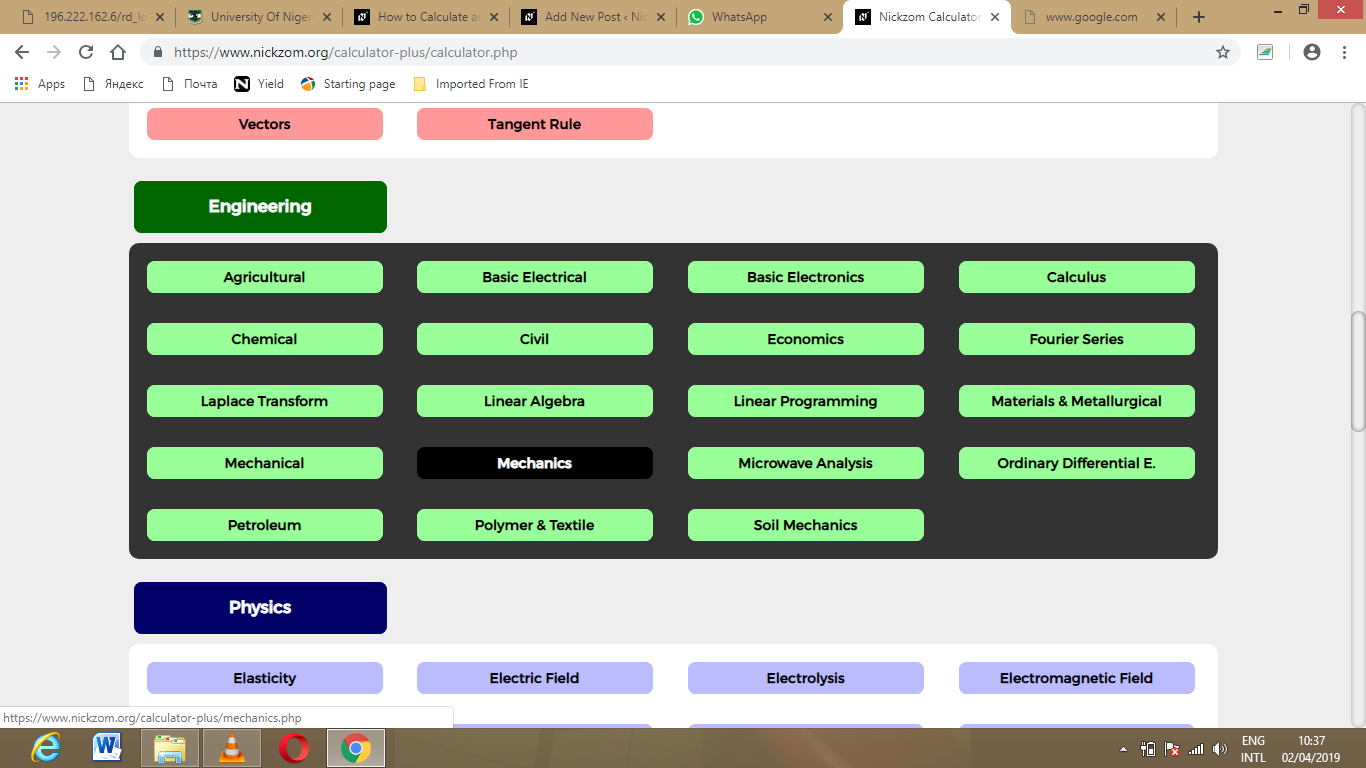Now, Click on Motion of Circular Path under Mechanics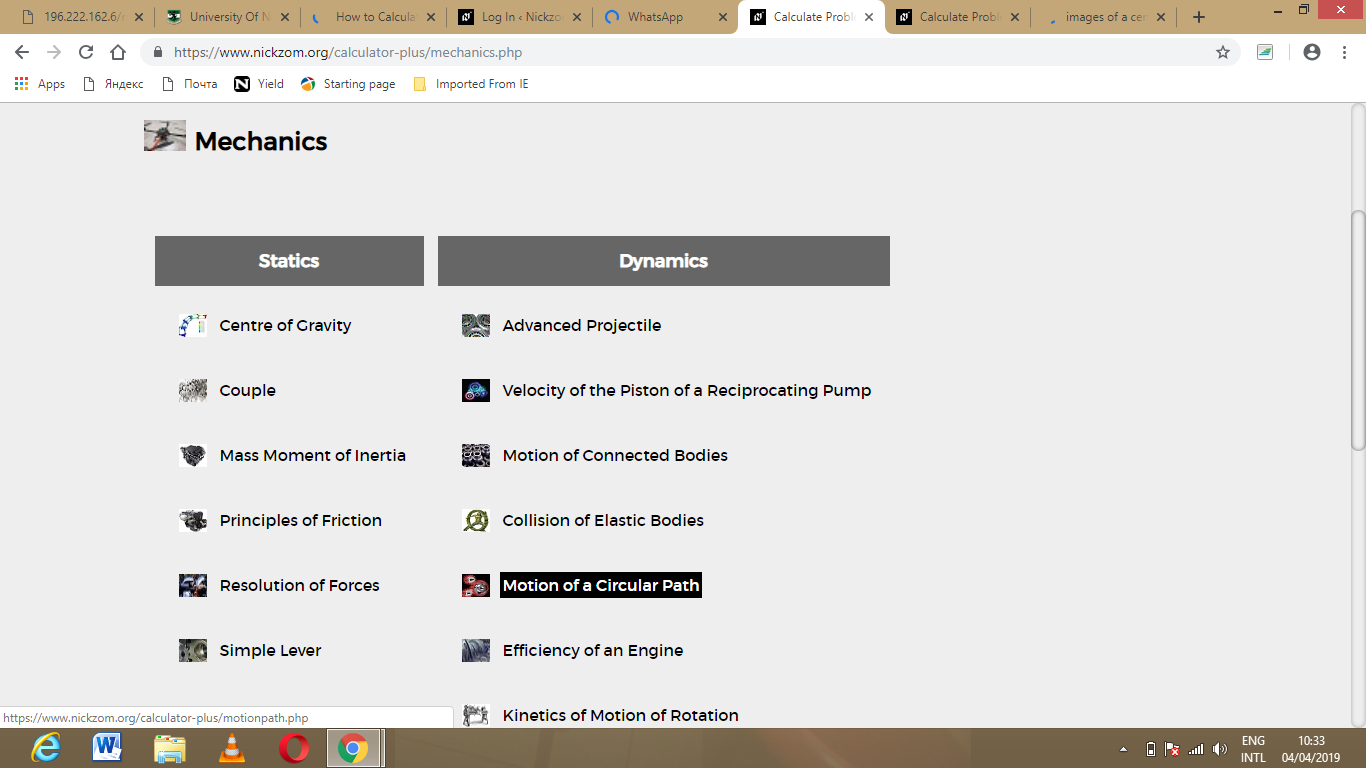Click on Reaction of the inner wheel of a vehicle moving along a level circular path under Motion of Circular Path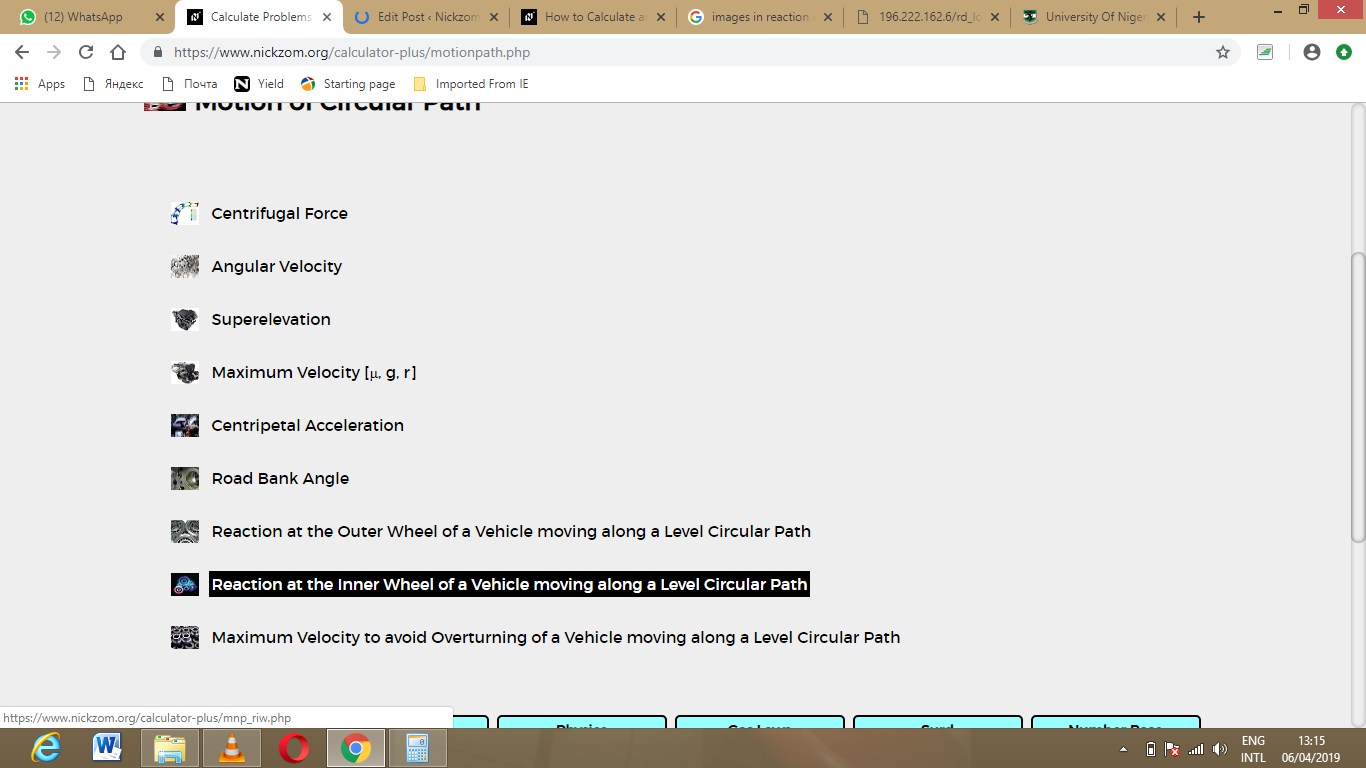The screenshot below displays the page or activity to enter your value, to get the answer for the reaction of the inner wheel of a vehicle moving along a level circular path according to the respective parameter which are the Mass of the Vechicle (m), Acceleration due to Gravity (g), Velocity of the Vehicle (v), Height of Centre of Gravity of the Vehicle from Ground Level (h), Radius of Circular Path (r) and Half of the Distance between the Centre Lines of the Wheel (a).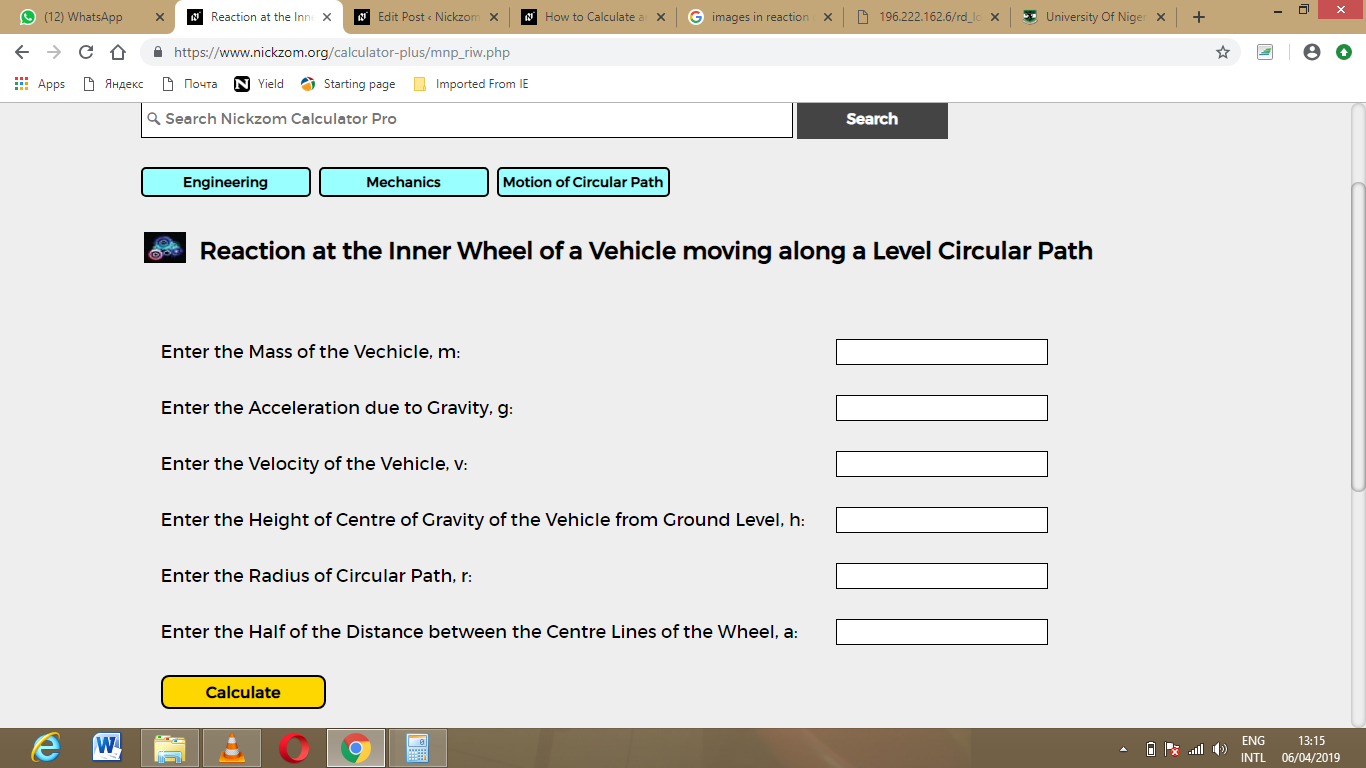Now, enter the value appropriately and accordingly for the parameter as required by the Mass of the Vechicle (m) is 13Acceleration due to Gravity (g) is 9.8Velocity of the Vehicle (v) is 11, Height of Centre of Gravity of the Vehicle from Ground Level (h) is 5Radius of Circular Path (r) is 7 and Half of the Distance between the Centre Lines of the Wheel (a) is 3.Finally, Click on Calculate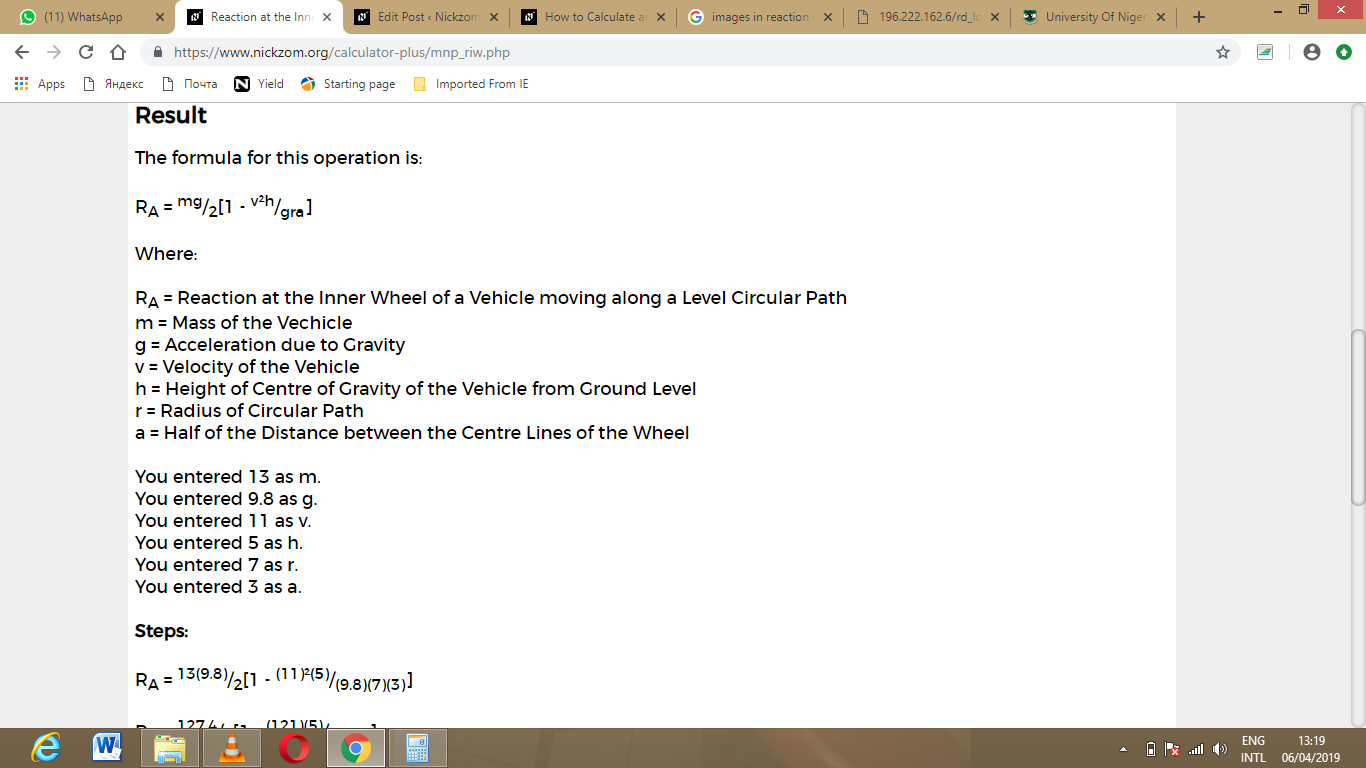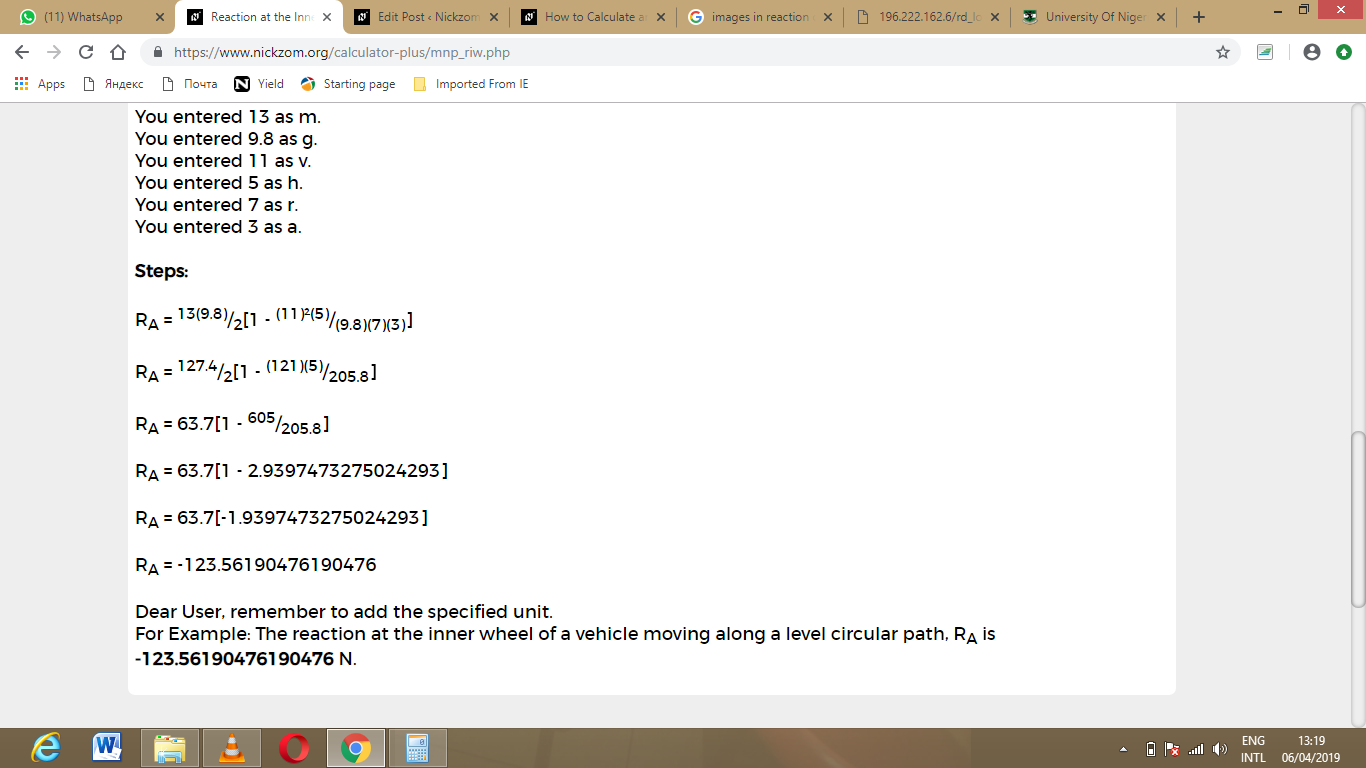As you can see from the screenshot above, Nickzom Calculator – The Calculator Encyclopedia solves for the reaction of the inner wheel of a vehicle moving along a level circular path and presents the formula, workings and steps too.